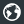Λέσχη Φίλων Στατιστικής - GrStats forumJoin the forum, it's quick and easy

Λέσχη Φίλων Στατιστικής - GrStats forumΛέσχη Φίλων Στατιστικής - GrStats forum
Would you like to react to this message? Create an account in a few clicks or log in to continue.
Για προβλήματα εγγραφής και άλλες πληροφορίες επικοινωνήστε με : grstats.forum@gmail.com ή grstats@stat-athens.aueb.gr

# Poker probability (Texas hold 'em)Posts : 364
Join date : 2009-10-17
Location : Utrecht, The Netherlands##Poker probability (Texas hold 'em)

Mon 8 Feb 2010 - 16:35
Το έβαλα στον αθλητισμό.. Παιχνίδι δεν είναι;! Λιγότερο κουραστικό ακόμα και από τα Darts(Βελάκια) βέβαια! Αλλά περισσότερο ενδιαφέρον στατιστικά..

In poker, the probability of many events can be determined by direct calculation. This article discusses computing probabilities for many commonly occurring events in the game of Texas hold 'em and provides some probabilities and odds for specific situations. In most cases, the probabilities and odds are approximations due to rounding.

When calculating probabilities for a card game such as Texas Hold 'em, there are two basic approaches. The first approach is to determine the number of outcomes that satisfy the condition being evaluated and divide this by the total number of possible outcomes. For example, there are six outcomes (ignoring order) for being dealt a pair of aces in Hold' em: {A, A}, {A, A}, {A, A}, {A, A}, {A, A}, and {A, A}. There are 52 ways to pick the first card and 51 ways to pick the second card and two ways to order the two cards yielding (52×51)/2=1326 possible outcomes when being dealt two cards (also ignoring order). This gives a probability of being dealt two aces of \begin{matrix} \frac{6}{1326} = \frac{1}{221} \end{matrix}.

The second approach is to use conditional probabilities, or in more complex situations, a decision tree. There are 4 ways to be dealt an ace out of 52 choices for the first card resulting in a probability of \begin{matrix} \frac{4}{52} = \frac{1}{13} \end{matrix}. There are 3 ways of getting dealt an ace out of 51 choices on the second card after being dealt an ace on the first card for a probability of \begin{matrix} \frac{3}{51} = \frac{1}{17} \end{matrix}. The conditional probability of being dealt two aces is the product of the two probabilities: \begin{matrix} \frac{1}{13} \times \frac{1}{17} = \frac{1}{221} \end{matrix}.

Often, the key to determining probability is selecting the best approach for a given problem. This article uses both of these approaches.
Εδώ για το άρθρο στα αγγλικά.. http://en.wikipedia.org/wiki/Poker_probability_%28Texas_hold_%27em%29

Here are the probabilities and odds of being dealt various other types of starting hands.Περισσότερα εδώ : http://en.wikipedia.org/wiki/Texas_hold_%27em_starting_hands
Εδώ για υπολογισμό των odds με τη χρήση εφαρμογής : http://gr.pokernews.com/poker-tools/poker-odds-calculator.htm
Permissions in this forum:
You cannot reply to topics in this forum